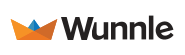on March 23, 2020

# Dynamic text color based on background

TL;DR: If you want your text color to change to black or white dynamically according to background color contrast, you can use the following code:

``````function getRGB(c) {
return parseInt(c, 16) || c
}

function getsRGB(c) {
return getRGB(c) / 255 <= 0.03928
? getRGB(c) / 255 / 12.92
: Math.pow((getRGB(c) / 255 + 0.055) / 1.055, 2.4)
}

function getLuminance(hexColor) {
return (
0.2126 * getsRGB(hexColor.substr(1, 2)) +
0.7152 * getsRGB(hexColor.substr(3, 2)) +
0.0722 * getsRGB(hexColor.substr(-2))
)
}

function getContrast(f, b) {
const L1 = getLuminance(f)
const L2 = getLuminance(b)
return (Math.max(L1, L2) + 0.05) / (Math.min(L1, L2) + 0.05)
}

function getTextColor(bgColor) {
const whiteContrast = getContrast(bgColor, '#ffffff')
const blackContrast = getContrast(bgColor, '#000000')

return whiteContrast > blackContrast ? '#ffffff' : '#000000'
}``````

## See it in action

Here is a pen showing how this works. Just click the button to get a random background color and text color will change accordingly.

## How this could be useful

On a project I’m working on, there is a page customized with brand colors of our customers. A component uses primary brand color as background color and places a text on top of it. The problem is setting a single good text color is impossible, because whatever I pick might just not have enough contrast with the background. So I came up with this solution to make the text work with any brand color.

## Math behind it

To calculate the contrast between two colors, we’ll use the following contrast ratio formula, defined by WCAG guidelines:

``(L1 + 0.05) / (L2 + 0.05)``

Here L1 and L2 are relative luminance values of our two (foreground and background) colors. Relative luminance is a measure of how bright a color is perceived to the human eye1 and it has a formula of:

``Y = 0.2126R + 0.7152G + 0.0722B``

Where R, G, B are sRGB components of a color. So to get sRGB components of a hex color we need some helper functions.

``````function getRGB(c) {
return parseInt(c, 16) || c
}

function getsRGB(c) {
return getRGB(c) / 255 <= 0.03928
? getRGB(c) / 255 / 12.92
: Math.pow((getRGB(c) / 255 + 0.055) / 1.055, 2.4)
}

function getLuminance(hexColor) {
return (
0.2126 * getsRGB(hexColor.substr(1, 2)) +
0.7152 * getsRGB(hexColor.substr(3, 2)) +
0.0722 * getsRGB(hexColor.substr(-2))
)
}``````

Using the relative luminance of each color, we can get the contrast between them:

``````function getContrast(f, b) {
const L1 = getLuminance(f)
const L2 = getLuminance(b)
return (Math.max(L1, L2) + 0.05) / (Math.min(L1, L2) + 0.05)
}``````

Finally, we can calculate two sets of contrast - between our color, and black or white - to determine which combination is more visible.

``````function getTextColor(bgColor) {
const whiteContrast = getContrast(bgColor, '#ffffff')
const blackContrast = getContrast(bgColor, '#000000')

return whiteContrast > blackContrast ? '#ffffff' : '#000000'
}``````

## More examples out there

Chrome DevTools is using this method to get the contrast ratio between the color of any DOM element and it’s background. Also, it rates the contrast based on WCAG-defined levels.

### WebAIM

WebAIM has an online contrast checker that shows contrast between two colors and whether it passes minimum WCAG levels.

1. ^

To our eyes, green appears brightest while blue appears the least bright.

### Mentions